# Riemannian domain

(diff) ← Older revision | Latest revision (diff) | Newer revision → (diff)

Riemann domain, complex (-analytic) manifold overAn analogue of the Riemann surface of an analytic functionof a single complex variablefor the case of analytic functions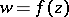,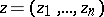, of several complex variables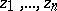,.

More precisely, a path-connected Hausdorff spaceis called an (abstract) Riemann domain if there is a local homeomorphism (a projection)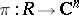such that for each pointthere is a neighbourhoodthat transforms homeomorphically into a polydisc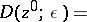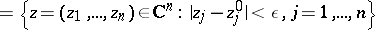in the complex space. A Riemann domain is a separable space.

A complex functionis called holomorphic onif for any point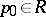the function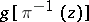ofcomplex variablesis holomorphic in the corresponding polydisc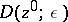. The projectionis given by the choice ofholomorphic functions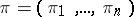, which correspond to coordinatesin. Starting from a given regular element of an analytic function, its Riemann domain is constructed in the same way as the Riemann surface of a given analytic function of one complex variable, i.e. initially by means of analytic continuation one constructs the complete analytic function, and then, using neighbourhoods, one introduces a topology into the set of elements of the complete analytic function. Like Riemann surfaces, Riemann domains arise unavoidably in connection with analytic continuation of a given element of an analytic function when, following the ideas of B. Riemann, one tries to represent the complete analytic functionas a single-valued point function on a domain.

In particular, Riemann domains arise as multi-sheeted domains of holomorphy of analytic functions of several complex variables. Oka's theorem states that a Riemann domain is a domain of holomorphy if and only if it is holomorphically convex (see Holomorphically-convex complex space).

Modern studies of Riemann domains are conducted within the framework of the general theory of analytic spaces. A generalization of the concept of a domain of holomorphy leads to Stein spaces (cf. Stein space).

How to Cite This Entry:
Riemannian domain. Encyclopedia of Mathematics. URL: http://encyclopediaofmath.org/index.php?title=Riemannian_domain&oldid=12703
This article was adapted from an original article by E.D. Solomentsev (originator), which appeared in Encyclopedia of Mathematics - ISBN 1402006098. See original article